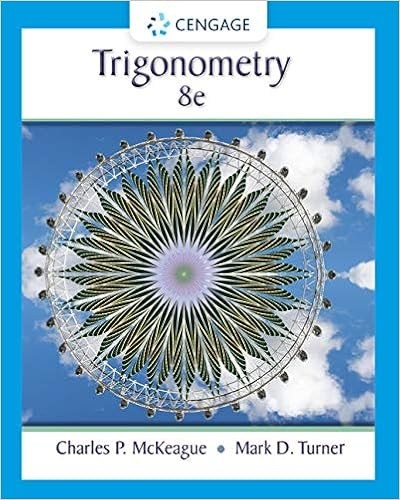# AP_Physics_Topic_5_Student_Notes.doc - Topic 5 Conservation...

• Notes
• 19

This preview shows page 1 - 3 out of 19 pages.

##### We have textbook solutions for you!
The document you are viewing contains questions related to this textbook.The document you are viewing contains questions related to this textbook.
Chapter 1 / Exercise 2
Trigonometry
McKeague/TurnerExpert Verified
Topic 5 Conservation of Linear MomentumI.Introduction.
II.Conservation of Linear Momentum.
.
ii.d.The total momentum of a system of particles is the vector sum of the momenta of the individual particles.i.1
##### We have textbook solutions for you!
The document you are viewing contains questions related to this textbook.The document you are viewing contains questions related to this textbook.
Chapter 1 / Exercise 2
Trigonometry
McKeague/TurnerExpert Verified
Topic 5 Conservation of Linear Momentumii.But according to Newton’s second law for a system of particles, equals the net external force acting on the system.iii.e.When the sum of the external forces acting on a system of particles remains zero, the rate of change of the total momentum remains zero and the total momentum of the system remains constant.i.ii.This result is known as the law of conservation of momentum.iii.iv.This law is one of the most important in physics. It is more widely applicable than the law of conservation of mechanicalenergy because internal forces exerted by one particle in a system on another are oftennot conservative. The nonconservative internal forces can change the total mechanical energy of the system, though they effect no change of the system’s total momentum. If the total momentum of a system remains constant, then the velocity of the center of mass of the systemremains constant. The law of conservation of momentum is a vector relation, so it is valid component by component.f.Finding Velocities Using Momentum Conservation (Equation 8-5)i.PICTUREDetermine that the net external force (or ) on the system is negligible for some interval of time. If the net force is determined not to be negligible, do not proceed.
•••